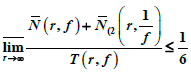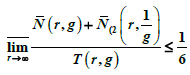#### 期刊菜单

Uniqueness Theorem of Entire Functions with Share on Value IM
DOI: 10.12677/PM.2021.111011, PDF , HTML, XML, 下载: 323  浏览: 424

Abstract: In this paper, we shall prove that if two non-constant entire function f(z) and g(z) share the value 1 IM, and,, then f(z)≡g(z) or f(z)·g(z)≡1.

1. 引言及主要结果

1976年，M. Ozuwa证明了如下定理：

1987年，仪洪勋证明了：

$\underset{r\to +\infty }{\stackrel{¯}{\mathrm{lim}}}\frac{\stackrel{¯}{N}\left(r,f\right)+{\stackrel{¯}{N}}_{\left(2}\left(r,\frac{1}{f}\right)}{T\left(r,f\right)}\le \frac{1}{6}$, (1.1)

$\underset{r\to +\infty }{\stackrel{¯}{\mathrm{lim}}}\frac{\stackrel{¯}{N}\left(r,g\right)+{\stackrel{¯}{N}}_{\left(2}\left(r,\frac{1}{g}\right)}{T\left(r,g\right)}\le \frac{1}{6}$, (1.2)

$f\left(z\right)\equiv g\left(z\right)$$f\left(z\right)\cdot g\left(z\right)\equiv 1$

2. 几个引理

$N\left(r,\frac{1}{{f}^{\left(k\right)}}\right).

${\stackrel{¯}{N}}_{L}\left(r,\frac{\text{1}}{f-1}\right)\le \stackrel{¯}{N}\left(r,f\right)+S\left(r,f\right)$, ${\stackrel{¯}{N}}_{L}\left(r,\frac{\text{1}}{g-1}\right)\le \stackrel{¯}{N}\left(r,g\right)+S\left(r,g\right)$.

$\underset{r\to \infty }{\stackrel{¯}{\mathrm{lim}}}\frac{\stackrel{¯}{N}\left(r,f\right)+{\stackrel{¯}{N}}_{\left(2}\left(r,\frac{1}{f}\right)}{T\left(r,f\right)}\le \frac{1}{\text{2}}$, $\underset{r\to \infty }{\stackrel{¯}{\mathrm{lim}}}\frac{\stackrel{¯}{N}\left(r,g\right)+{\stackrel{¯}{N}}_{\left(2}\left(r,\frac{1}{g}\right)}{T\left(r,g\right)}\le \frac{1}{2}$,

$f\left(z\right)\equiv g\left(z\right)$$f\left(z\right)\cdot g\left(z\right)\equiv 1$

3. 定理1的证明

$H=\left(\frac{{f}^{″}}{{f}^{\prime }}-\frac{{g}^{″}}{{g}^{\prime }}\right)-2\left(\frac{{f}^{\prime }}{f-1}-\frac{{g}^{\prime }}{g-1}\right)$.

${N}_{E}^{1\right)}\left(r,\frac{1}{f-1}\right)\le N\left(r,\frac{1}{H}\right)\le T\left(r,H\right)+O\left(1\right)\le N\left(r,H\right)+S\left(r,f\right)+S\left(r,g\right)$. (1.3)

$N\left(r,H\right)\le {\stackrel{¯}{N}}_{\left(2}\left(r,\frac{1}{f}\right)+{\stackrel{¯}{N}}_{\left(2}\left(r,\frac{1}{g}\right)+{\stackrel{¯}{N}}_{L}\left(r,\frac{1}{f-1}\right)+{\stackrel{¯}{N}}_{L}\left(r,\frac{1}{g-1}\right)+{N}_{0}\left(r,\frac{1}{{f}^{\prime }}\right)+{N}_{0}\left(r,\frac{1}{{g}^{\prime }}\right)$. (1.4)

$\stackrel{¯}{N}\left(r,\frac{1}{f-1}\right)=\stackrel{¯}{N}\left(r,\frac{1}{g-1}\right)\le {N}_{E}^{1\right)}\left(r,\frac{1}{f-1}\right)+{N}_{E}^{\left(2}\left(r,\frac{1}{f-1}\right)+{\stackrel{¯}{N}}_{L}\left(r,\frac{1}{f-1}\right)+{\stackrel{¯}{N}}_{L}\left(r,\frac{1}{g-1}\right)$. (1.5)

$\begin{array}{l}\stackrel{¯}{N}\left(r,\frac{1}{f-1}\right)+\stackrel{¯}{N}\left(r,\frac{1}{g-1}\right)\\ \le \text{2}{N}_{E}^{1\right)}\left(r,\frac{1}{f-1}\right)+\text{2}{N}_{E}^{\left(2}\left(r,\frac{1}{f-1}\right)+\text{2}{\stackrel{¯}{N}}_{L}\left(r,\frac{1}{f-1}\right)+\text{2}{\stackrel{¯}{N}}_{L}\left(r,\frac{1}{g-1}\right)\\ \le {\stackrel{¯}{N}}_{\left(2}\left(r,\frac{1}{f}\right)+{\stackrel{¯}{N}}_{\left(2}\left(r,\frac{1}{g}\right)+{\stackrel{¯}{N}}_{L}\left(r,\frac{1}{f-1}\right)+{\stackrel{¯}{N}}_{L}\left(r,\frac{1}{g-1}\right)+{N}_{0}\left(r,\frac{1}{{f}^{\prime }}\right)+{N}_{0}\left(r,\frac{1}{{g}^{\prime }}\right)\\ \text{\hspace{0.17em}}\text{\hspace{0.17em}}+{N}_{E}^{1\right)}\left(r,\frac{1}{f-1}\right)+\text{2}{N}_{E}^{\left(2}\left(r,\frac{1}{f-1}\right)+\text{2}{\stackrel{¯}{N}}_{L}\left(r,\frac{1}{f-1}\right)+\text{2}{\stackrel{¯}{N}}_{L}\left(r,\frac{1}{g-1}\right)+S\left(r,f\right)+S\left(r,g\right)\end{array}$. (1.6)

$\begin{array}{l}{N}_{E}^{\text{1}\right)}\left(r,\frac{1}{f-1}\right)+\text{2}{N}_{E}^{\left(2}\left(r,\frac{1}{f-1}\right)+{\stackrel{¯}{N}}_{L}\left(r,\frac{1}{f-1}\right)+{\stackrel{¯}{N}}_{L}\left(r,\frac{1}{g-1}\right)\\ \le \frac{\text{1}}{\text{2}}\left(N\left(r,\frac{1}{f-1}\right)+N\left(r,\frac{1}{g-1}\right)\right)\le \frac{\text{1}}{\text{2}}\left(T\left(r,f\right)+T\left(r,g\right)\right)+O\left(1\right)\end{array}$.(1.7)

$\begin{array}{l}\stackrel{¯}{N}\left(r,\frac{1}{f-1}\right)+\stackrel{¯}{N}\left(r,\frac{1}{g-1}\right)\\ \le {\stackrel{¯}{N}}_{\left(2}\left(r,\frac{1}{f}\right)+{\stackrel{¯}{N}}_{\left(2}\left(r,\frac{1}{g}\right)+2{\stackrel{¯}{N}}_{L}\left(r,\frac{1}{f-1}\right)+2{\stackrel{¯}{N}}_{L}\left(r,\frac{1}{g-1}\right)+{N}_{0}\left(r,\frac{1}{{f}^{\prime }}\right)\\ \text{\hspace{0.17em}}\text{\hspace{0.17em}}+{N}_{0}\left(r,\frac{1}{{g}^{\prime }}\right)+\frac{\text{1}}{\text{2}}\left(T\left(r,f\right)+T\left(r,g\right)\right)+S\left(r,f\right)+S\left(r,g\right)\end{array}$.(1.8)

$\begin{array}{l}T\left(r,f\right)+T\left(r,g\right)\\ \le \stackrel{¯}{N}\left(r,\frac{1}{f-1}\right)+\stackrel{¯}{N}\left(r,\frac{1}{g-1}\right)+\stackrel{¯}{N}\left(r,\frac{1}{f}\right)+\stackrel{¯}{N}\left(r,\frac{1}{g}\right)\\ \text{\hspace{0.17em}}\text{\hspace{0.17em}}-{N}_{0}\left(r,\frac{1}{{f}^{\prime }}\right)-{N}_{0}\left(r,\frac{1}{{g}^{\prime }}\right)+S\left(r,f\right)+S\left(r,g\right)\end{array}$. (1.9)

$\begin{array}{l}\frac{\text{1}}{\text{2}}T\left(r,f\right)+\frac{1}{2}T\left(r,g\right)\\ \le 3\stackrel{¯}{N}\left(r,\frac{1}{f}\right)+3\stackrel{¯}{N}\left(r,\frac{1}{g}\right)+{\stackrel{¯}{N}}_{\left(2}\left(r,\frac{1}{f}\right)+{\stackrel{¯}{N}}_{\left(2}\left(r,\frac{1}{g}\right)+S\left(r,f\right)+S\left(r,g\right)\\ \le 3\left[\stackrel{¯}{N}\left(r,\frac{1}{f}\right)+{\stackrel{¯}{N}}_{\left(2}\left(r,\frac{1}{f}\right)\right]+3\left[\stackrel{¯}{N}\left(r,\frac{1}{g}\right)+{\stackrel{¯}{N}}_{\left(2}\left(r,\frac{1}{g}\right)\right]+S\left(r,f\right)+S\left(r,g\right)\end{array}$.(1.10)

$\stackrel{¯}{N}\left(r,\frac{1}{f}\right)+{\stackrel{¯}{N}}_{\left(2}\left(r,\frac{1}{f}\right)<\left(\frac{1}{6}-\epsilon \right)T\left(r,f\right)$, $\stackrel{¯}{N}\left(r,\frac{1}{g}\right)+{\stackrel{¯}{N}}_{\left(2}\left(r,\frac{1}{g}\right)<\left(\frac{1}{6}-\epsilon \right)T\left(r,g\right)$. (1.11)

$\text{6}\epsilon \left(T\left(r,f\right)+T\left(r,g\right)\right)\le S\left(r,f\right)+S\left(r,g\right)$.

  杨乐. 值分布论及其新研究[M]. 北京: 科学出版社, 1982.  仪洪勋, 杨重骏. 亚纯函数唯一性理论[M]. 北京: 科学出版社, 1995.  王丽. 具有一个分担值集和亏量的整函数的一个性质[J]. 楚雄师范学院学报, 2016(12): 8-13.  Ozawa, M. (1976) Unicity Theorems for Entire Functions. Journal d’Analyse Mathématique, 30, 411-420. https://doi.org/10.1007/BF02786728  仪洪勋. 具有两个亏值的亚纯函数[J]. 数学学报, 1987(30): 588-597.  林秀清, 仪洪勋. 分担1IM公共值的整函数的唯一性定理[J]. 数学进展, 2011, 40(1): 79-86.  Ye, S.Z. (1992) Uniqueness of Meromorphic Funcitions That Share Three Values. Kodai Mathematical Journal, 15, 236-243.